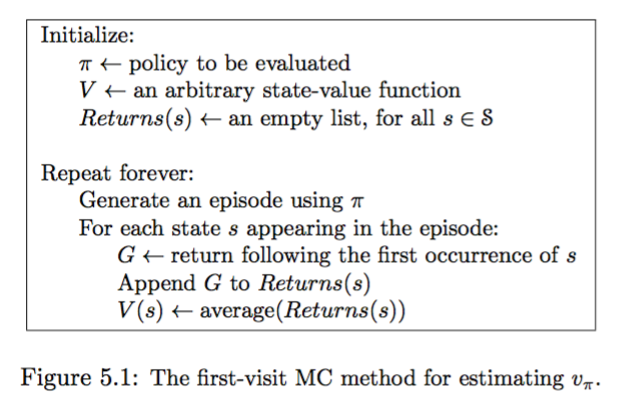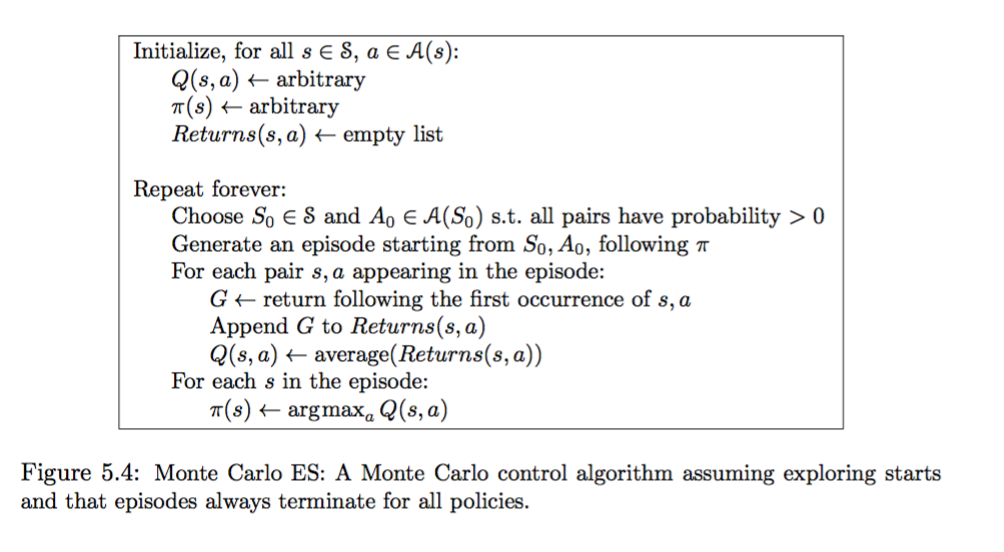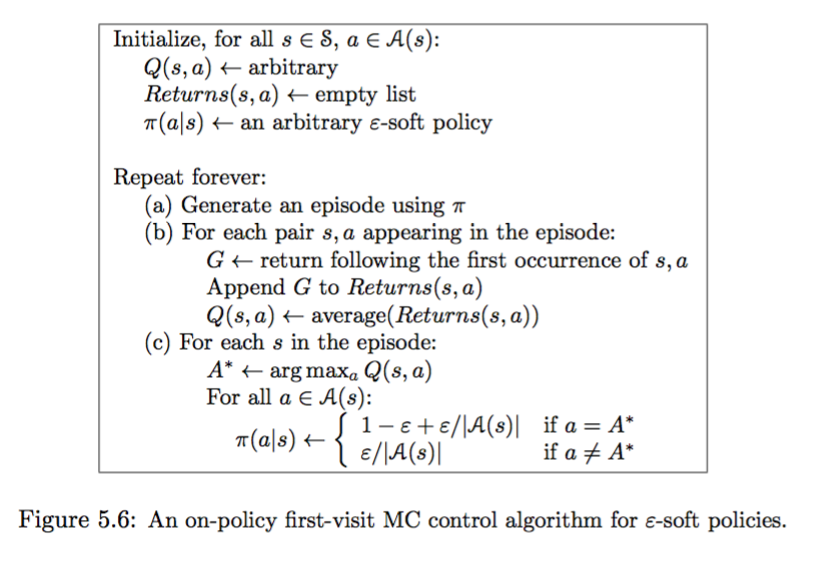Spencer Lyon

# Monte Carlo methods

· by Spencer Lyon · Read in about 3 min · (494 Words)
MC RL algorithms

This is part 2 in the reinforcement learning for economists notes. For part 1 see Reinforcement Learning Intro. For a list of all entries in the series go here

## Monte Carlo methods

As defined in Reinforcement Learning Intro GPI encompasses the core ideas for all the RL algorithms in this book. One family of such algorithms is Monte Carlo (MC) methods. One distinguishing feature of these methods is that they can be implemented in a completely model-free way. This means that to apply them we need to know nothing about the dynamics of the model; we simply need to be able to observe sequences of states, actions, and rewards.

### Prediciton methods

Monte Carlo prediciton is a method for learning the state-value function for a given policy. The algorithm is given by:Notice that the MC prediction algorithm does not update any estimates of the value function until after the entire episode is realized. In this sense we would say that the method does not bootstrap, meaning it does not use approximations of the value in other states to update the value in a particular state (value iteration is bootstrapping).

### Control methods

Monte Carlo control is an iterative algorithm where each iteration contains a Monte Carlo prediction step followed by a policy improvment step. The improvement step is simply done by making the policy greedy with the predicted value function.

Some technical conditions must be satisfied to ensure convergence of this naive algorithm:

• episodes have exploring starts (meaning that they don’t attempt to be greedy or optimal at the start)
• There are an infinite number of episodes

These are ok theoretically, but prohibative computationally.

Monte Carlo ES is an algorithm that does away with the assumption that you need an infinite number of episodes. It proceeds asNotice that we don’t need the assumption of an infinite number of episodes because we don’t do a full policy evaluation each step.

To do away with the exploring starts assumption we need to define a few more terms.

• A policy $\pi$ is said to be $\varepsilon$-soft if $\pi(a|s) > \frac{\varepsilon}{| A(s) |}$ for all $a \in A$ and $s \in S$.
• An $\varepsilon$-greedy is a policy where all non-greedy actions are chosen with probability $\frac{\varepsilon}{| A(s) |}$, while the greedy policy is chosen with probability $1 - \varepsilon + \frac{\varepsilon}{| A(s) |}$.
• On-policy methods evaluate and improve the policy that is used to make decisions.
• Off-policy methods use one policy to make decisions while trying to evaluate and improve another policy.
• A first-visit MC method uses only the return (not reward) after the first occurance of a state (or state-action pair) when doing evaluation. An every-visit method uses the return after every occurance of a state (or state-action pair) when doing evaluation.

An on-policy first-visit MC control algorithm for $\varepsilon$-soft policies is given below:### Importance sampling

This section will include more prediction and control methods, but is an important enough concept that it deserves its own section.